TIKZ package

Created by Jianfeng Chen. Contact jchen37@ncsu.edu

Introduction

Tikz is a Latex package to draw charts. This tutorial is using the following packages.
You should include them at the beginning.

\usepackage{graphicx}
\usepackage{subfig}
\usepackage{amsmath}
\usepackage{tikz}
\usepackage{pgfplots}
\usetikzlibrary{shapes,arrows}
\usepgfplotslibrary{statistics}  % for the boxplot stats
\usepackage{pgf-pie} % for pie chart. download from https://ctan.org/pkg/pgf-pie?lang=en  unzip and put pgf-pie.sty into project

More examples here!

Plot scatter

Data should be express as csv format
and them hard code the csv into latex before \begin{document}.

\begin{filecontents*}{detla_pd.csv}
X,Y1,Y2,Y3
1,0,0,0
2,0,0,0
3,0,0,0
4,4.7,0,11.1
5,4.7,0.1,11.9
6,5.5,11.1,14.2
7,5.5,11.1,14.2
8,5.6,14.2,16.6
9,5.6,16.6,16.6
10,9.5,16.6,16.6
11,9.5,16.6,16.7
12,9.5,16.6,19
13,11.9,16.6,19.1
14,14.2,16.7,21.4
15,14.3,19,21.4
16,14.3,19.1,22.2
17,14.7,21.4,22.2
18,16.7,22.2,26.2
19,16.7,23.8,28.5
20,16.7,27.7,33.3
21,16.7,28.5,33.4
22,16.7,33,33.6
23,16.7,33.4,36.5
24,21.4,33.6,38.1
25,22.2,33.9,38.9
26,22.6,42.8,42.8
27,23.8,42.8,42.8
28,25.2,42.8,42.8
29,27.7,42.8,42.8
30,28.5,50,50
\end{filecontents*}

To reduce the complexity of main.tex, you can put the filecontents* block in separate file and use \input{xxx.tex} before \begin{document}

To draw a scatter, do the following

\begin{figure}[!]
\begin{tikzpicture}
\begin{axis}[
height=9cm,
width=8cm,
grid=major,
minor tick num=5,
title={Demonstration of tikz \LaTeX scatter},
xlabel={$x$-axis},
ylabel={$y$-axis},
legend style={at={(0.35,0.95)},anchor=north} % change legend location and style here
]
%\legend{} % add this if you don't need the legend
\end{axis}
\end{tikzpicture}
\caption{demo 1}
\end{figure}

Output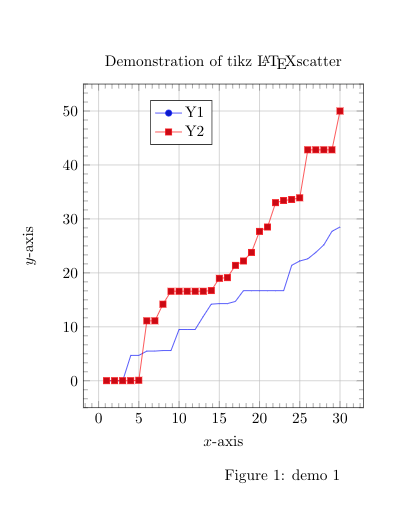Another example

\begin{tikzpicture}
\begin{axis}[title=Default arguments]
{2*x+3};
\end{axis}
\end{tikzpicture}

Output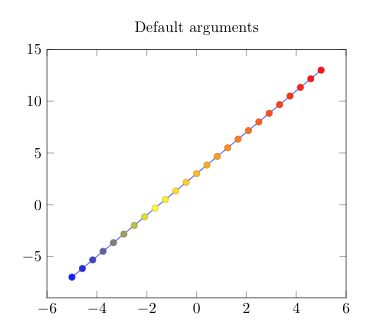Plot histogram

Create data first.
Again, filecontents* block must locate or inputed BEFORE \begin{document}

\begin{filecontents}{data.csv}
dist
1
2
2.5
2
1
3.5
3
1
3
2
1
1
0.5
1
1.5
1
\end{filecontents}

In the text

\begin{tikzpicture}
\begin{axis}[
ybar,
ymin=0
]
hist={
bins=7,
data min=0.5,
data max=4
}
] table [y index=0] {data.csv};
\end{axis}
\end{tikzpicture}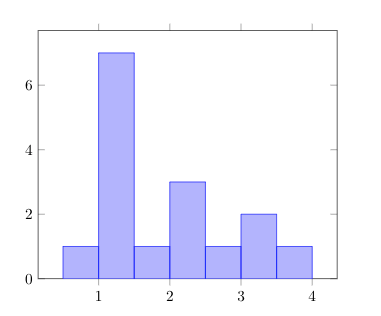Pie chart

% require the pgf-pie package
\begin{tikzpicture}
\pie [rotate = 180]
{62/Class A,
32/Class B, 6/Others}
\end{tikzpicture}

Output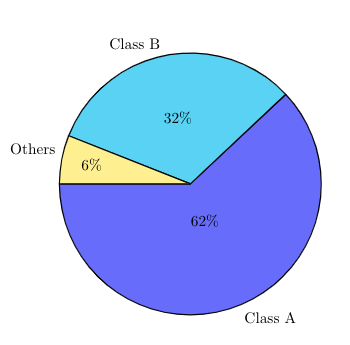More for pie chart at http://latex-cookbook.net/articles/pie-chart/

Boxplot

\begin{tikzpicture}
\begin{axis}[
boxplot/draw direction=y,
ylabel={time (s)},
height=8cm,
boxplot={
%
% Idea:
%  place the
%  group 1 at 0,2 + 0,4 + 0,6 + 0,8
%  group 2 at 1,2 + 1,4 + 1,6 + 1,8
%  group 3 at 2,2 + 2,4 + 2,6 + 2,8
%  group 3 at 3,2 + 3,4 + 3,6 + 3,8
%
% in a formular:
draw position={1/5 + floor(\plotnumofactualtype/4) + 1/5*mod(\plotnumofactualtype,4)},
%
% that means the box extend must be at most 0.2 :
box extend=0.15,
},
% ... it also means that 1 unit in x controls the width:
x=3cm,
% ... and it means that we should describe intervals:
xtick={0,1,2,...,50},
x tick label as interval,
xticklabels={%
{Data set 1},%
{Data set 2},%
},
x tick label style={
text width=2.5cm,
align=center
},
cycle list={{red},{blue},{green},{purple}},
]

table[row sep=\\,y index=0] {
data\\
60\\
516\\
710\\
503\\
1253\\
};
table[row sep=\\,y index=0] {
data\\
759\\
419\\
309\\
883\\
299\\
};
table[row sep=\\,y index=0] {
data\\
516\\
480\\
1356\\
200\\
736\\
};
table[row sep=\\,y index=0] {
data\\
684\\
340\\
700\\
325\\
377\\
};

table[row sep=\\,y index=0] {
data\\
60\\
516\\
710\\
503\\
1253\\
};
table[row sep=\\,y index=0] {
data\\
759\\
419\\
309\\
883\\
299\\
};
table[row sep=\\,y index=0] {
data\\
516\\
480\\
1356\\
200\\
736\\
};
table[row sep=\\,y index=0] {
data\\
684\\
340\\
700\\
325\\
377\\
};
\end{axis}
\end{tikzpicture}

Output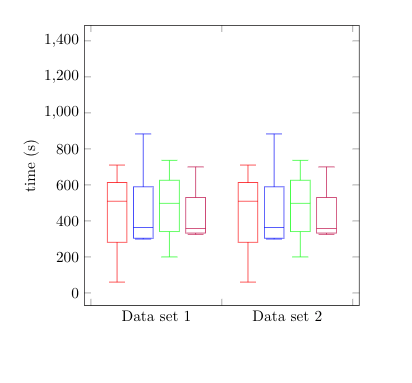As you can see, in the above example, the data is embedded in the text. Obviously, we can put that in separate file.

Block diagram

\pgfdeclarelayer{background}
\pgfdeclarelayer{foreground}
\pgfsetlayers{background,main,foreground}
\tikzstyle{sensor}=[draw, fill=blue!20, text width=5em,  text centered, minimum height=2.5em]
\tikzstyle{ann} = [above, text width=5em]
\tikzstyle{naveqs} = [sensor, text width=6em, fill=red!20, minimum height=12em, rounded corners]
\def\blockdist{2.3}
\def\edgedist{2.5}
\begin{tikzpicture}
\path (naveq.140)+(-\blockdist,0) node (gyros) [sensor] {Gyros};
\path (naveq.-150)+(-\blockdist,0) node (accel) [sensor] {Accelero-meters};

\path [draw, ->] (gyros) -- node [above] {$\alpha$}  (naveq.west |- gyros) ;
\path [draw, ->] (accel) -- node [above] {$\beta$}  (naveq.west |- accel);
\node (IMU) [below of=accel] {IMU};
\path (naveq.south west)+(-0.6,-0.4) node (INS) {INS};

\begin{pgfonlayer}{background}
\path (gyros.west |- naveq.north)+(-0.5,0.3) node (a) {};
\path (INS.south -| naveq.east)+(+0.3,-0.2) node (b) {};
\path[fill=yellow!20,rounded corners, draw=black!50, dashed]
(a) rectangle (b);
\path (gyros.north west)+(-0.2,0.2) node (a) {};
\path (IMU.south -| gyros.east)+(+0.2,-0.2) node (b) {};
\path[fill=blue!10,rounded corners, draw=black!50, dashed]
(a) rectangle (b);
\end{pgfonlayer}
\end{tikzpicture}

Output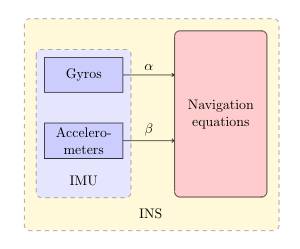Clone this wiki locally
You can’t perform that action at this time.